# If the barrier to rotation around C-C bond of bromoethane is 3.6 kcal mol/mol calculate the...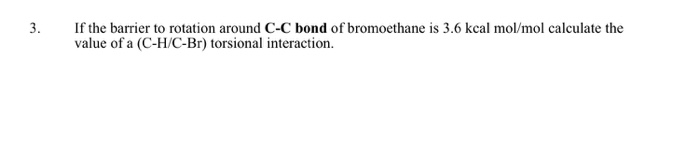If the barrier to rotation around C-C bond of bromoethane is 3.6 kcal mol/mol calculate the value of a (C-H/C-Br) torsional interaction.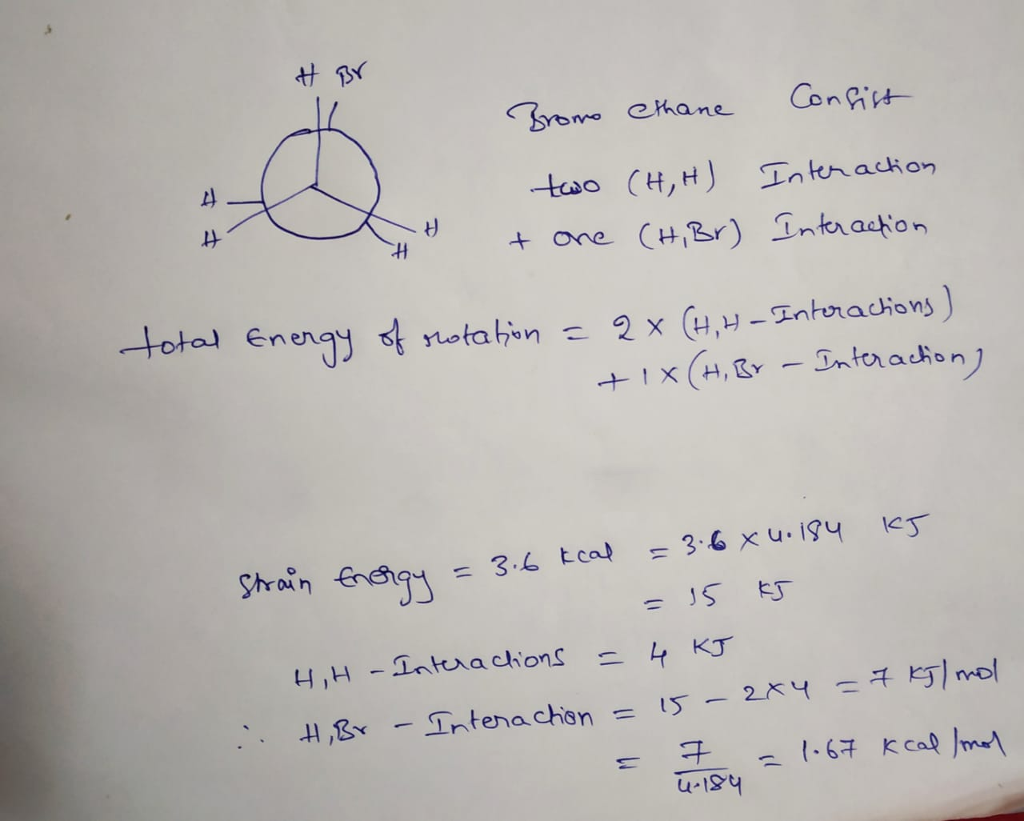#### Earn Coin

Coins can be redeemed for fabulous gifts.

Similar Homework Help Questions
• ### Question 6 (2 points) Consider the rotation around the C2-C3 bond of 2-methylbutane. Using the values...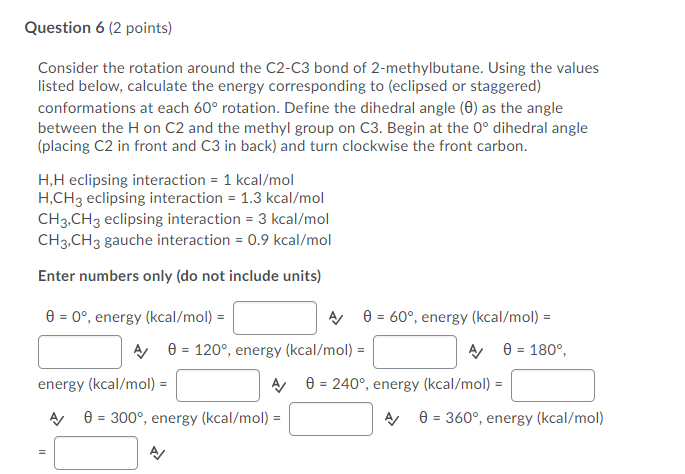Question 6 (2 points) Consider the rotation around the C2-C3 bond of 2-methylbutane. Using the values listed below, calculate the energy corresponding to (eclipsed or staggered) conformations at each 60° rotation. Define the dihedral angle (6) as the angle between the H on C2 and the methyl group on C3. Begin at the O° dihedral angle (placing C2 in front and C3 in back) and turn clockwise the front carbon. H,H eclipsing interaction = 1 kcal/mol H,CH3 eclipsing interaction =...

• ### Assuming that the bond dissociation energy of the C-H bond is 550.0 kJ mol-1 (131.5 kcal...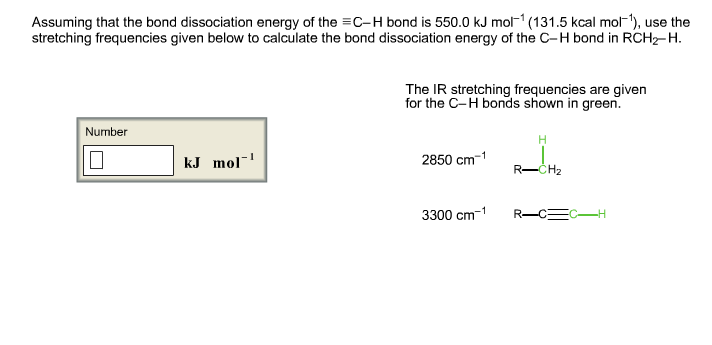Assuming that the bond dissociation energy of the C-H bond is 550.0 kJ mol-1 (131.5 kcal mol-1), use the stretching frequencies given below to calculate the bond dissociation energy of the C-H bond in RCH2-H. The IR stretching frequencies are given for the C-H bonds shown in green.

• ### REPORT SHEET FOR CONFORMATIONAL ANALYSIS OF ALKANES AND CYCLOALKANES Ethane caMMFF Calculated Energies (kcal/mole) from Property...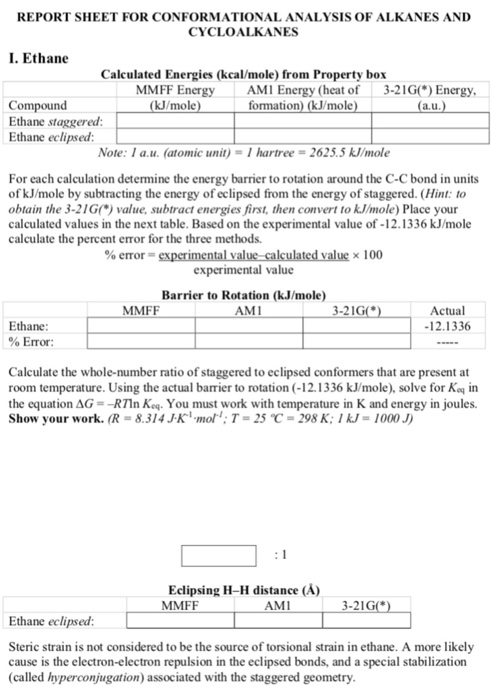REPORT SHEET FOR CONFORMATIONAL ANALYSIS OF ALKANES AND CYCLOALKANES Ethane caMMFF Calculated Energies (kcal/mole) from Property box MMFF Energy AMI Energy (heat of 3-21GO Energy kJ/mole Compound Ethane staggered Ethane eclipsed: formation) (kJ/mole a.u Note: I a.u. (atomic unit)-I hartree 2625.5 kJ/mole For each calculation determine the energy barrier to rotation around the C-C bond in units of kJ/mole by subtracting the energy of eclipsed from the energy of staggered. (Hint: to obtain the 3-21G) value, subtract energies first, then...

• ### 6. Conformational Analysis: Newman Projections (25 points) Consider the rotation around the C3-C4 bond of (3R...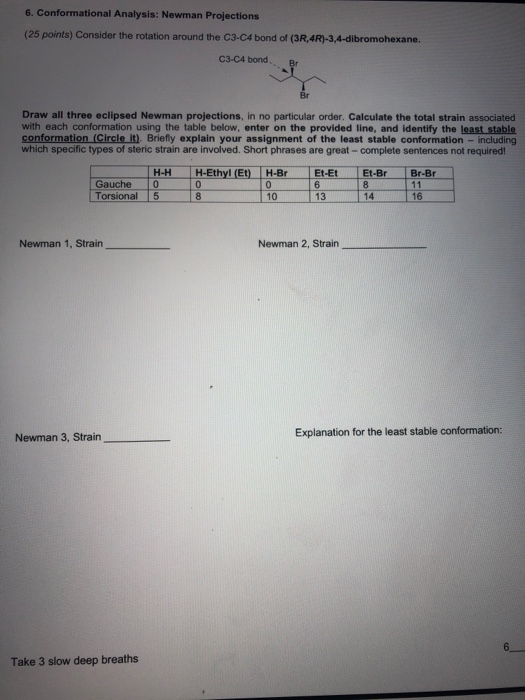6. Conformational Analysis: Newman Projections (25 points) Consider the rotation around the C3-C4 bond of (3R 4R)-3,4-dibromohexane. C3-C4 bond. B Draw all three eclipsed Newman projections. In no particular order. Calculate the total strain associated with each conformation using the table below, enter on the provided line, and identify the least stable conformation (Circle it) Briefly explain your assignment of the least stable conformation - including which specific types of steric strain are involved. Short phrases are great complete sentences...

• ### TABLE 6.1 BOND DISSOCIATION ENERGIES (A) OF COMMON BONDS KJ/MOL KCAL/MOL Bonds to H H2C=CH-CH2 HCC-CH,...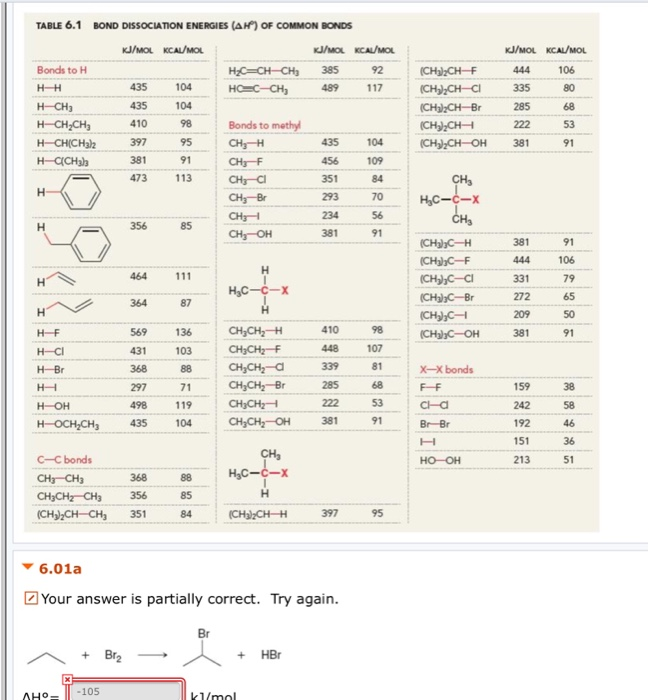TABLE 6.1 BOND DISSOCIATION ENERGIES (A) OF COMMON BONDS KJ/MOL KCAL/MOL Bonds to H H2C=CH-CH2 HCC-CH, KJ/MOL KCAL/MOL 385 92 489117 HH 435 80 104 104 KJ/MOL KCAL/MOL 444 106 335 285 222 381 91 (CH3)2CH-F (CH) CH-CI (CH3)2CHBr (CH),CH (CH3)2CH-OH H-CH3 H-CH2CH3 HCH(CH3 H- CH3) 435 104 109 Bonds to methyl CH; CHEF CH3-C1 CH3-Br CH3 CH, OH CH I I 356 85 381 91 HC-c-X CHE (CH3)3C-H (CH3),C-F (CH),C-C1 (CH3)2C-Br (CH3), (CH)C-OH 381 444 331 106 111 464...

• ### How would I calculate an SN1 activation Barrier when I am given the enthalpies of formation?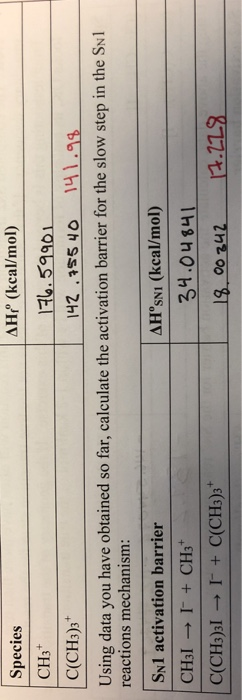how would I calculate an SN1 activation Barrier when I am given the enthalpies of formation? AHP (kcaV/mol) Species CH3 C(CH3)3 Using data you have obtained so far, calculate the activation barrier for the slow step in the Swl 142 55 4o 141.99 reactions mechanism: SN1 activation barrier ΔHoSNI (kcal/mol) 34.04 841 C(CH3)3l I+ C(CH3)3 00 242 4.119 AHP (kcaV/mol) Species CH3 C(CH3)3 Using data you have obtained so far, calculate the activation barrier for the slow step in the...

• ### For each of the following compounds, predict the energy barrier to rotation (looking down any one of the C—C bonds). Dra...

For each of the following compounds, predict the energy barrier to rotation (looking down any one of the C—C bonds). Draw a Newman projection and then compare the staggered and eclipsed conformations. Remember that we assigned 4 kJ/mol to each pair of eclipsing H’s and 6 kJ/mol to an H eclipsing a methyl group: (a) 2,2-Dimethylpropane (b) 2-Methylpropane

• ### Ho for the pre c! . Given the bond dissociation energies below in Kcal/mol, estimate the ano forth (CH),CH-CI step...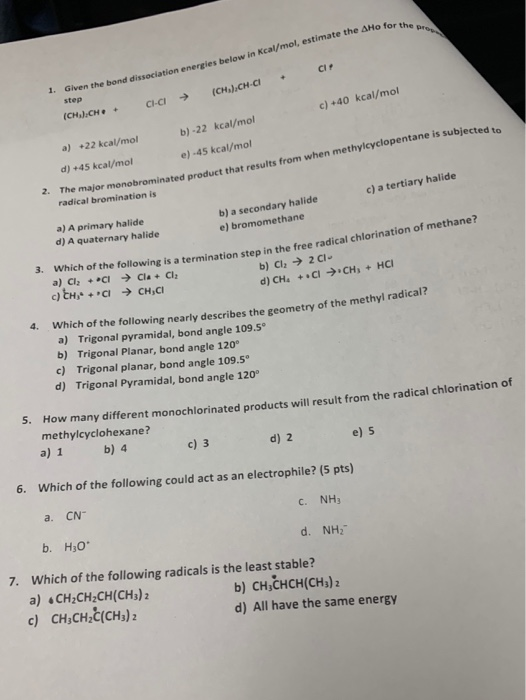Ho for the pre c! . Given the bond dissociation energies below in Kcal/mol, estimate the ano forth (CH),CH-CI step . CHCI c) 40 kcal/mol (CH.J.CH a) +22 kcal/mol b)-22 kcal/mol e) -45 kcal/mol he major monobrominated product that results from when methylcyclopentane is subjected d) +45 kcal/mol c) a tertiary halide radical bromination is b) a secondary halide e) bromomethane a) A primary halide d) A quaternary halide 3. Which of the following is a termination a) Cl +...

• ### Question 1a. (0.5 point) Consider the bond dissociation energies listed below in kcal/mol CH3 – Br...

Question 1a. (0.5 point) Consider the bond dissociation energies listed below in kcal/mol CH3 – Br 70 CH3CH2 – Br 68 (CH3)2CH – Br 68 (CH3)3C – Br 65 These data show that the carbon–bromine bond is weakest when bromine is bound to a ____. a) methyl carbon b) primary carbon c) secondary carbon d) tertiary carbon e) quaternary carbon 1b. Predict the product for the halogenation reaction given below when methyl cyclohexane is subjected to free radical bromination Br2...

• ### Bond Dissociation Energies (for A-B Bond broken AH, kJ/mol Bond broken → A A4, kJ/mol +...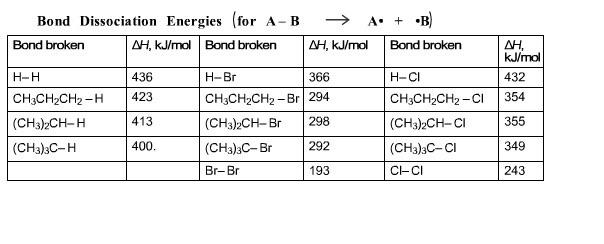Bond Dissociation Energies (for A-B Bond broken AH, kJ/mol Bond broken → A A4, kJ/mol + B) Bond broken mo H-H CH3CH2CH2-H (CH3)2CH-H (CH3)3C-H 436 423 413 400. H-Br 366 CH3CH2CH2-Br 294 (CH3)2CH-Br 298 (CH3)3C-Br292 Br-Br 193 H-CI 432 CH3CH2CH2-CI 354 (CH3)2CH-CI 355 (CH3)3C-CI 349 CI-CI 243 Alkane halogenation is a two-step reaction, as shown below. Using the table of bond dissociation energies, calculate the enthalpy of each step and the enthalpy of the overall reaction. Step 1: Number CH3CHCH3...